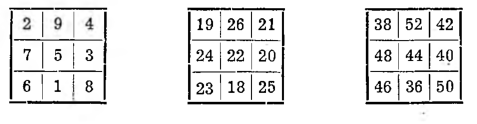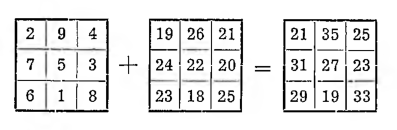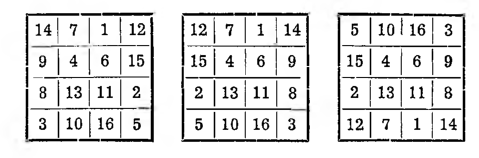# Division of magic squares

Magic figures are divided into flat and spatial, for there are squares, triangles, rectangles, polygons and magic circles, but there are also magic cubes.

Squares divide: depending on progress, in which numbers go - for arithmetic and geometric; depending on the side scales - odd (3, 5, 7, 9 and so on), oddly–Even (6, 10, 14, 18 and so on) and even-even (4, 8, 12, 16 and so on); finally, depending on the setting of the numbers in the square - to magic ordinary, magical with special properties, hypermagic.

The magic square will remain magic, if all the numbers, which it contains, we will enlarge or reduce by one and the same number. It will also remain magical, when we multiply or divide all its components by some constant. For a clear understanding, it is enough to present it with one example:There is a magic sum in the first square, that is, the sum of the numbers of the individual rows, columns or diagonals, is 15; in the second square we add to each number po 17 and the magic sum is 15 + 3 • 17 = 66; and finally in the third square we multiply all terms by 2 and the magic sum is 2 • 66 = 132.

II. If the square is magic for some arithmetic progress, it will be magical for the same distributed arithmetic progress with a different prime and a different difference. Yes, for example. in the first of the given magic squares instead of numbers:

1, 2, 3, 4, 5, 6, 7, 8, 9 you can arrange the words of progress accordingly:

91, 96, 101, 106, 111, 116, 121, 126, 131.

From all these rules, an extremely important practical tip can be drawn, that when forming any magic square it is enough to put it forward from the simplest numbers, so from the numbers of a natural sequence: 1, 2, 3, 4, 5, . .., because then by multiplication, division, increasing or decreasing these numbers you can achieve an infinite number of magic squares with the most diverse magic sums.

Another extremely important property of magic squares is this, that from two squares we can get the third by summing the numbers standing in the corresponding fields:The magic sum of such a square is equal to the sum of the magic sums of both components: 81 = 15 + 66.

The square will not lose its magic, if we rearrange its columns and rows symmetrically to the center of the square. For example:In the first of these squares, we have rearranged the first and fourth columns; the second square was created, with the sum of the words in each row and column, but the sum of the diagonals was not preserved. Now if we rearrange the first and fourth lines in the second square, then we get the third square, already perfectly magical.